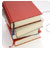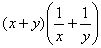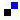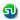### CAT, XAT, TANCET, FMS, SNAP, Sample Problems. MBA Entrance Preparation Questions Ascent Education : TANCET Study Material

 Home TANCET Classes XAT '16 Class SAT Classes TANCET Study Material CAT '16 Classes
 TANCET ClassesWeekend and weekday TANCET MBA and MCA classes @ Chennai. Ascent students state toppers. Centers at Anna Nagar, Nungambakkam and Velachery.XAT Coaching ClassesWeekend, weekday and crash courses for XAT @ Chennai. Faculty includes IIM, XIM Bhubaneswar graduates. Course material and mock XATs provided.Other Courses GMAT Classes GRE Classes SAT Classes TANCET Classes & Courses Other Links Ascent TANCET Toppers Testimonials MBA Entrance Question bank Business Partnerships Careers @ Ascent Contact Us +91 44 4500 8484 +91 96000 48484 ascent@ascenteducation.com Postal Address
You are here: Home  »   CAT, TANCET Sample Problems  »  August 2003

# TANCET, XAT, CAT Sample Problems : August 2003

1. August 04, 2003 : Number Theory ›  When a number is divided by 36, it leaves a remainder of 19. What will be the remainder when the number is divided by 12? Solution »

2. August 05, 2003 ›  Mark 1 If the question can be answered by using one of the statements alone, but cannot be answered by using the other statement alone.
Mark 2 If the question can be answered by using either statement alone.
Mark 3 If the question can be answered by using both statements together, but cannot be answered by using either statement alone.
Mark 4 If the question cannot be answered even by using both the statements together.

Is x = y?
A.= 4
B. (x - 50)2 = (y - 50)2 Solution »

3. August 06, 2003 ›  A zookeeper counted the heads of the animals in a zoo and found it to be 80. When he counted the legs of the animals he found it to be 260. If the zoo had either pigeons or horses, how many horses were there in the zoo? Solution »

4. August 07, 2003 : Number Properties ›  Let x, y and z be distinct integers. x and y are odd and positive, and z is even and positive. Which one of the following statements cannot be true? Solution »

5. August 08, 2003 ›  A red light flashes 3 times per minute and a green light flashes 5 times in two minutes at regular intervals. If both lights start flashing at the same time, how many times do they flash together in each hour? Solution »

6. August 11, 2003 : Number Properties ›  Anita had to do a multiplication. Instead of taking 35 as one of the numbers to be multiplied, she took it as 53. As a result, the product increased by 540. What is the incorrect product? Solution »

7. August 12, 2003 : Averages ›  Three math classes: X, Y, and Z, take an algebra test.
The average score in class X is 83.
The average score in class Y is 76.
The average score in class Z is 85.
The average score of all students in classes X and Y together is 79.
The average score of all students in classes Y and Z together is 81.

What is the average for all the three classes? Solution »

8. August 13, 2003 ›  Three runners A, B and C run a race, with runner A finishing 12 meters ahead of runner B and 18 meters ahead of runner C, while runner B finishes 8 meters ahead of runner C. Each runner travels the entire distance at a constant speed.

What was the length of the race? Solution »

9. August 14, 2003 : Number Theory ›  Let n be the number of different 5 digit numbers, divisible by 4 with the digits 1, 2, 3, 4, 5 and 6, no digit being repeated in the numbers. What is the value of n? Solution »

10. August 18, 2003 ›  What is the circum radius of a triangle whose sides are 7, 24 and 25 respectively? Solution »

11. August 19, 2003 : Arithmetic Mean ›  When a student weighing 45 kgs left a class, the average weight of the remaining 59 students increased by 200g. What is the average weight of the remaining 59 students? Solution »

12. August 20, 2003 ›  In how many ways can 15 people be seated around two round tables with seating capacities of 7 and 8 people? Solution »

13. August 21, 2003 : Coordinate Geometry ›  What is the equation of the line that is parallel to the line 3x + 7y = 10 and passes through the point (4, 8)? Solution »

14. August 22, 2003 ›  Yana and Gupta leave points x and y towards y and x respectively simultaneously and travel in the same route. After meeting each other on the way, Yana takes 4 hours to reach her destination, while Gupta takes 9 hours to reach his destination. If the speed of Yana is 48 km/hr, what is the speed of Gupta? Solution »

## CAT, XAT, TANCET Practice Questions and Answers : Listed Topicwise

 Number Theory Permutation Combination Probability Inequalities Geometry Mensuration Trigonometry Data sufficiency Percentages Profit Loss Ratio Proportion Mixtures Alligation Speed Time Distance Pipes Cisterns Interest Races Average, Mean AP, GP, HP Set Theory Clocks Calendars Algebra Function English Grammar General AwarenessAdd to del.icio.usStumble Itdigg this